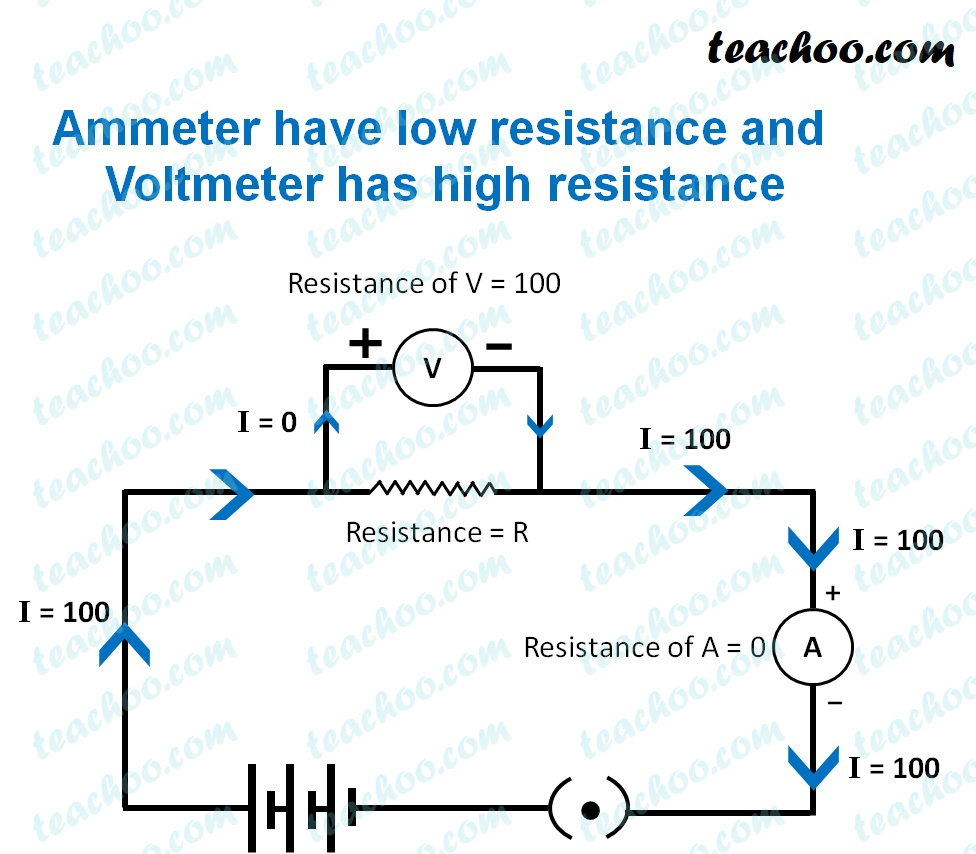Teachoo Questions

Class 10
Chapter 12 Class 10 - ElectricityAn ammeter is a device used to measure the amount of current flowing in a circuit.

The resistance of an ideal ammeter should be zero.

This is because an ammeter is connected in series in a circuit.

Hence, it's resistance adds to the total resistance of the circuit.

If the resistance of the ammeter would be high, the total resistance would be high.

This would decrease the amount of current flowing through the circuit.

Hence, in order to avoid the change of current flowing in a circuit.

The resistance of an ammeter should be as small as zero.

Learn in your speed, with individual attention - Teachoo Maths 1-on-1 Class